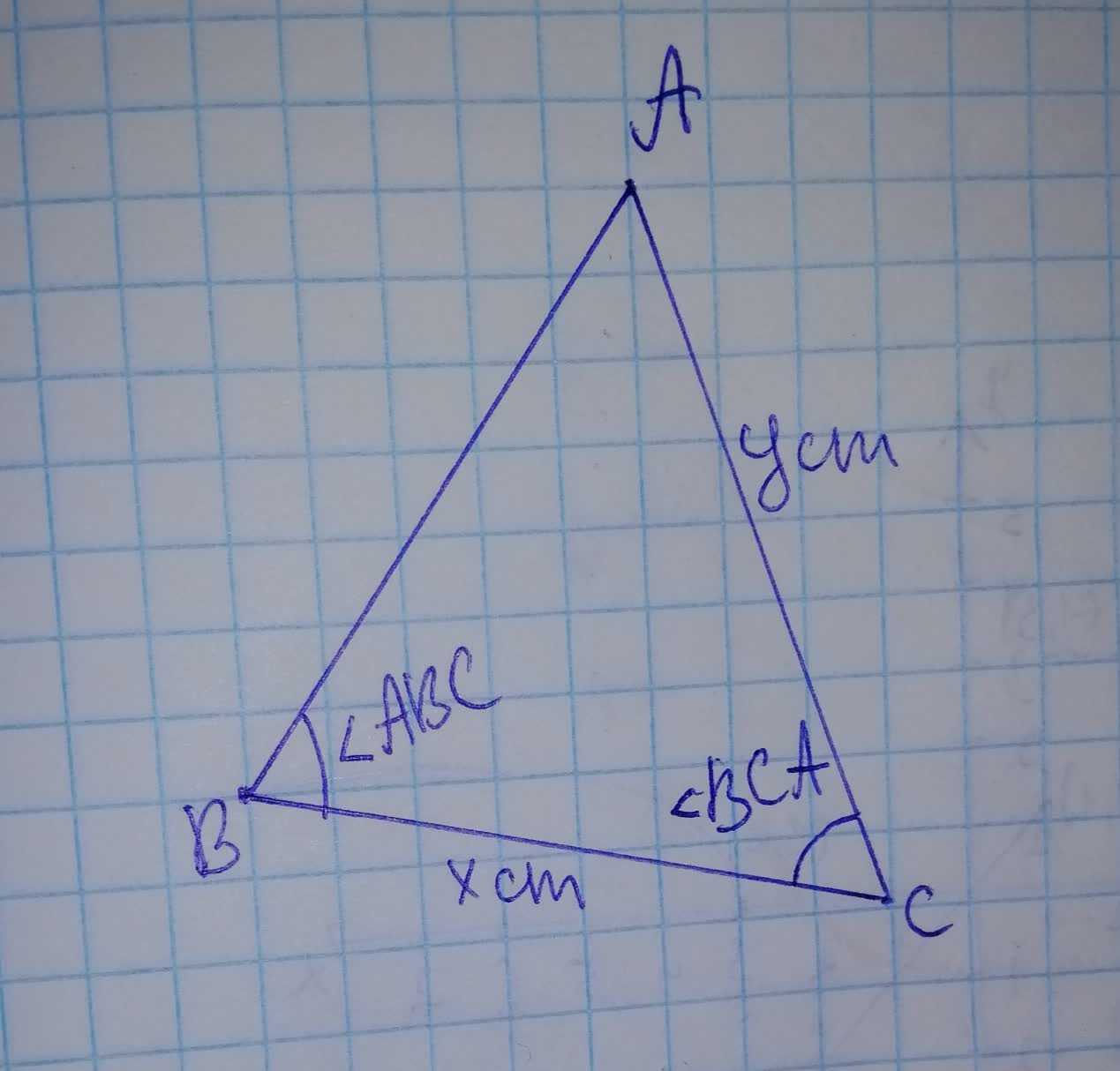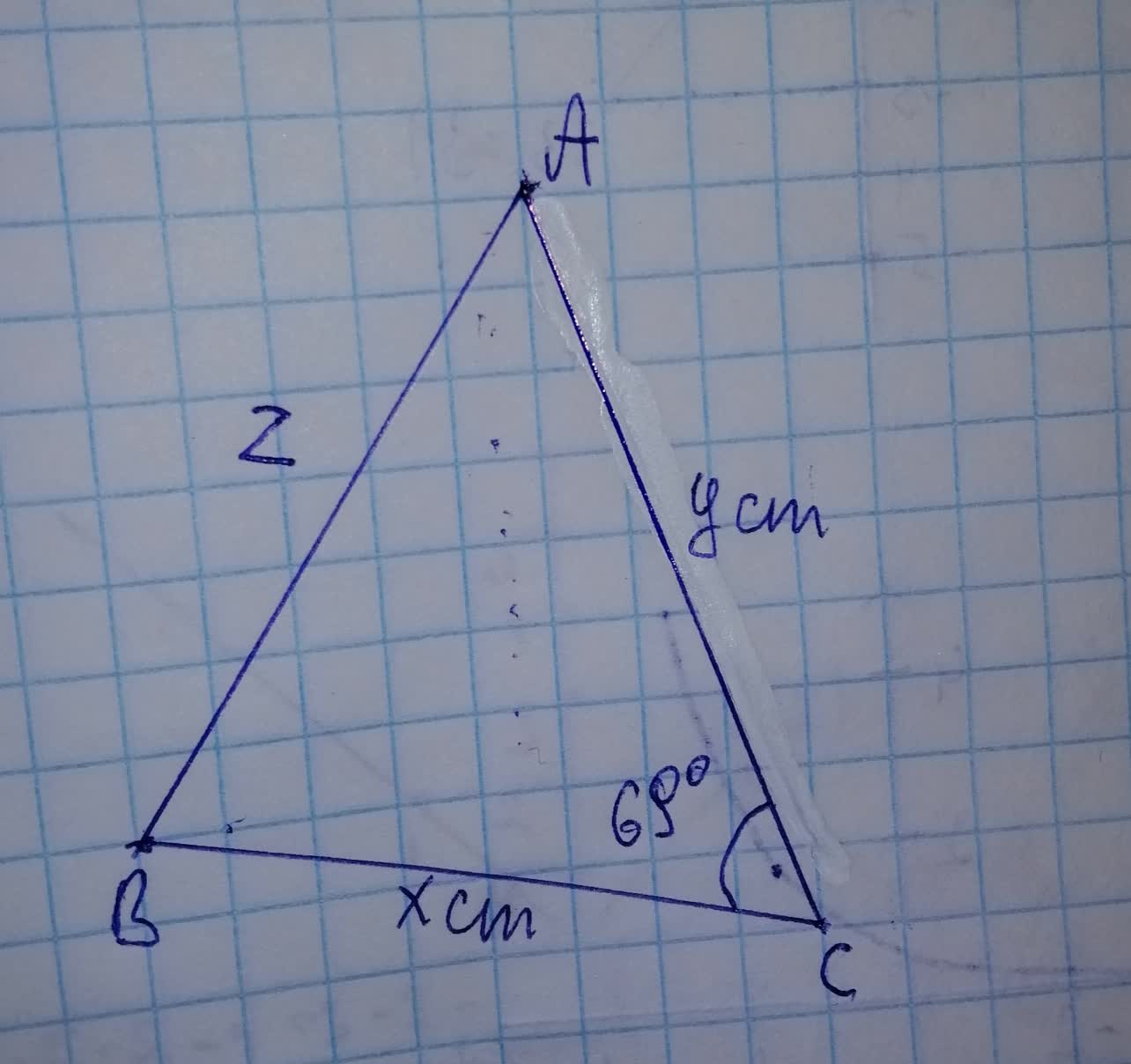# Consider the non-right triangle below 19610800141.jpg Suppose that m\angle BCA=69^{\circ}, and thatperomvu 2021-11-21 Answered
Consider the non-right triangle belowSuppose that $m\mathrm{\angle }BCA={69}^{\circ }$, and that $x=32cm$ and $y=49cm$. What is the degree measure of $\mathrm{\angle }ABC?$
You can still ask an expert for help

• Questions are typically answered in as fast as 30 minutes

Solve your problem for the price of one coffee

• Math expert for every subject
• Pay only if we can solve itJames Obrien

Step 1
Use cosine formula$\mathrm{\angle }BCA={69}^{\circ }$
$\mathrm{\angle }ABC=?$
${\mathrm{cos}69}^{\circ }⇒\frac{{x}^{2}+{y}^{2}-{2}^{2}}{2xy}$
$3583=\frac{{32}^{2}+{49}^{2}\cdot {2}^{2}}{2×32×49}$
$3563×2×32×49=$
$1123\cdot 8418=1024+2401-{2}^{2}$
$1123\cdot 8418=3425-{2}^{2}$
${z}^{2}⇒3425-1123\cdot 8418$
${z}^{2}=2301\cdot 1582$
$z=47\cdot 9703$
$\mathrm{\angle }\mathrm{cos}B=\frac{{z}^{2}+{x}^{2}-{y}^{2}}{22x}$
$\mathrm{\angle }\mathrm{cos}B=\frac{{\left(47\cdot 9703\right)}^{2}+{32}^{2}-{49}^{2}}{2×47\cdot 9703×32}$
Step 2
$\mathrm{cos}B=\frac{2301\cdot 1582+1024-2401}{3070.0992}$
$\mathrm{cos}B⇒\frac{924.1582}{3070.0992}$
$\mathrm{cos}B⇒\cdot 3010$
$\mathrm{\angle }ABC=B={\mathrm{cos}}^{-1}\left(\cdot 3010\right)$
$\mathrm{\angle }ABC={72.4811}^{\circ }$